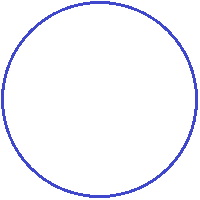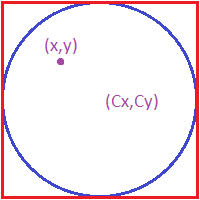## Tuesday, March 12, 2013

### Montecarlo method of finding the area of a circle and pi

You may know that the digits of pi look random but did you know that you can use randomness to find pi? You can actually approximate the area of any circle using random points in a method called the Montecarlo method. The principle of this method uses something called "sampling" which works as follows:

Say you want to find the number of people in a country who are female. You don't have time to check every single person so instead you take a random sample of people, say 10% of the population, and check only those. You find that 40% of the people in this sample are female so you generalize this percentage to the whole population and say that approximately 40% of the country is female. If the sample was random, this would be a reasonable approximation and this sort of thing is done all the time in surveys.

Now what we want to do is to find the area of a circle such as this:The Montecarlo method works in the following way:

Place that circle inside a square such as this:Keep in mind that the area of a square is easy to find. Now throw a point inside the square at random, which would result in something like this:Now if we repeat this process with many points and count how many of the points lie inside the circle, we can approximate what fraction of the area of the square is taken by the circle by treating the points as a sample of the total infinite number of points in the square.For example, in the above diagram there are 17 points in total but only 14 of the points lie inside the circle. So we can say that approximately 14/17 of the area inside the square is taken by the circle.

Now lets assume that we have a decent number of points and have a good approximation of the fraction of area that is taken by the circle. How can we use this fraction to find the area of the circle? Just multiply the fraction by the area in the square. Since we know the fraction of the area of the square that lies inside the circle, we can find the area of the circle by finding what this fraction of the area actually is.

So in the above example, the length of the side of the square is about 6cm, so the area of the square is 6x6 = 36cm^2, so the area of the circle is approximately 14/17 x 36 = 29.64cm^2.

In reality, since the circle has a radius which is half as big as the side of the square, its radius is 3cm, so its area is pi x 3^2 = 28.27cm^2. Not bad for a sample of 17 points which weren't exactly placed randomly.

How can this be used to find an approximation of pi? Just approximate the area of a circle of radius 1. The area would then be pi x 1^2 = pi.

Now let's use a computer to create lots of points. How can we check if a point is inside the circle using a computer? We can simply check if the distance of the point to the center of the circle is less than or equal to the radius of the circle. If the distance to the center is greater than the radius, than the point cannot be inside the circle.We find the distance of a point to the center by using Pythagoras' theorem as follows:Here is the Python 3 program we'll be using:
import math, random

def isPointInCircle(x, y, Cx, Cy, radius):
return math.sqrt((x - Cx)**2 + (y - Cy)**2) <= radius

pointsInside = 0
for i in range(numberOfPoints):
x = random.random()*squareSide
y = random.random()*squareSide

if (isPointInCircle(x, y, Cx, Cy, radius)):
pointsInside = pointsInside + 1

return pointsInside / numberOfPoints * squareSide**2

and we'll be finding what the area of a circle of radius 1 is for different numbers of points. Since the radius is 1, the area should approximate pi:
Number of pointsApproximate area (should be 3.14159...)
102.4
1003.0
10003.152
100003.14
100003.14896
1000003.14112